# KSEEB SSLC Class 10 Maths Solutions Chapter 7 Coordinate Geometry Ex 7.2

KSEEB SSLC Class 10 Maths Solutions Chapter 7 Coordinate Geometry Ex 7.2 are part of KSEEB SSLC Class 10 Maths Solutions. Here we have given Karnataka SSLC Class 10 Maths Solutions Chapter 7 Coordinate Geometry Exercise 7.2.

## Karnataka SSLC Class 10 Maths Solutions Chapter 7 Coordinate Geometry Exercise 7.2

Question 1.
Find the coordinates of the point which divides the join of (-1, 7) and (4, -3) in the ratio 2 : 3.
Solution:
Let P(x, y) is the required point.
P(x, y) divides the points A(-1, 7),
B (4, -3) in the ratio m1 : m2 = 2 : 3.
As per Section Formula, coordinates of P(x, y) areQuestion 2.
Find the coordinates of the points of trisection of the line segment joining (4, -1) and (-2, -3)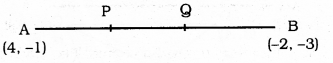Solution:
Let A(x1, y1) = A(4,-1)
B(x2, y2) = B(-2, -3).
P and Q are the points of trisection of AB.
AP = PQ = QB
i) P divides AB in the ratio m1 : m2 :: 1 : 2.
∴ As per Section Formula, Coordinates of P are :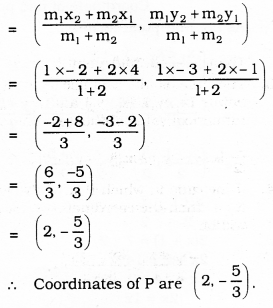ii) Q divides AB in the ratio 2 : 1.
∴ As per Section formula,Question 3.
To conduct Sports Day activities, in your rectangular shaped school ground ABCD, lines have been drawn with chalk powder at a distance of 1 m each. 100 flower pots have been placed at a distance of 1m from each other along AD, as shown in the following figure.Niharika runs $$\frac{1}{4}$$ th the distance of AD on then 2nd line and posts a green flag
Preet runs $$\frac{1}{5}$$ th the distance AD on the 5 eighthline and posts a red flag. What is the distance between both the flags ? If Rashmi has to post a blue flag exactly halfway between the line segment joining the two flags, where should she post her flag?
Solution:
Origin, A(0, 0)
AB along x – axis
In the parallel line AD in 2nd row, Rashmi sees green flag at the distance of $$\frac{1}{4}$$ .
∴ AD = $$\frac{1}{4}$$ × 100 = 25 m.
∴ Coordinates of green flag are G(2, 25)
Similarly we can find out coordinates of Red flag as R(8, 20).
∴ Distance between G and R is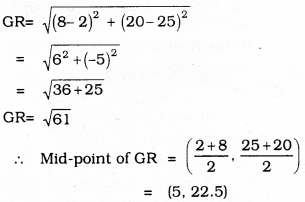It means blue flag is in 5th line and parallel to AD at the distance of 22.5 m.

Question 4.
Find the ratio in which the line segment joining the points (-3, 10) and (6, -8) is divided by (-1, 6).
Solution:
We have to check whether the line segment joining the points (-3, 10) and (6, -8) is divided by (-1, 6).
Let P(- 1, 6) divides AB in the ratio m1 : m2.
By using Section formula,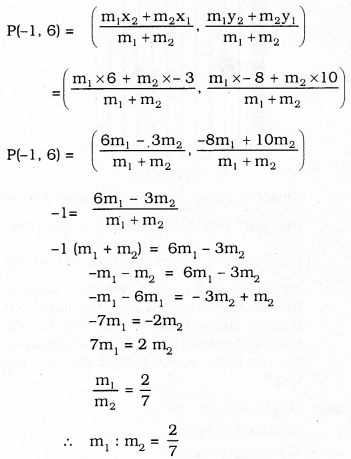∴ P(-1, 6) point divides the line segment in the ratio 2 : 7.

Question 5.
Find the ratio in whcih the line segment joining A(1, -5) and B(-4, 5) is divided by the x-axis. Also find the coordinates of the point of division.Solution:
Let the line segment joining A and B points divides x-axis in the ratio k : 1.
If coordinates of y, P(x, 0) compared,
$$\frac{k \times(5)+1 \times(-5)}{k+1}=0$$
5k – 5 = 0
∴ k = 1
∴ Ratio will be k : 1 = 1 : 1
∴ Point P(x, 0) is the mid-point of line segment AB.
∴ x = $$\frac{1-4}{2}=\frac{-3}{2}$$
∴ Dividing point, P($$-\frac{3}{2}$$, 1)

Question 6.
If (1, 2), (4, y), (x, 6) and (3, 5) are the vertices of a parallelogram taken in order, find x and y.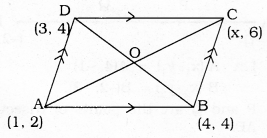Solution:
AC and BD are the diagonals of a parallelogram ABCD and diagonals bisect at O’.
∴ Coordinate of the midpoint of AC = Coordinate of midpoint BD.
As per Mid-point formula,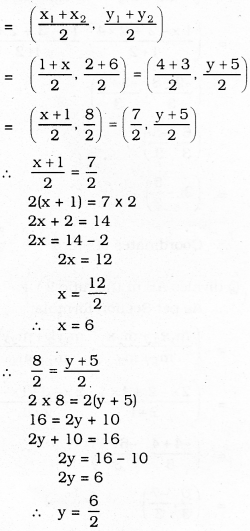∴ y = 3
∴ x = 6, y = 3.

Question 7.
Find the coordinates of point A, where AB is the diameter of a circle whose centre is (2, -3) and B is (1, 4).Solution:
AB is the diameter.
‘O’ is centre of the circle, bisects AB
Let coordinates of A are (x, y)
As per the Mid-point formula,∴ The coordinates of point A are (3, -10)

Question 8.
If A and B are (-2, -2) and (2, -4), respectively, find the coordinates of P such that AP = $$=\frac{3}{7}$$ AB and P lies on the line segment AB.Solution:
Let coordinates of P are (x, y).
AP = $$\frac{3}{7}$$ AB AP : AB = 3 : 7
As per Section formula,
AP : AB = 3 : 7.
∴ AP + PB = AB
3 + PB = 7
∴ PB = = 7 – 3 = 4
∴ Let AP : PB = 3 : 4 = m1 : m2.
Coordinates of P are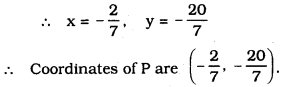Question 9.
Find the coordinates of the points which divide the line segment joining A(-2, 2) and B(2, 8) into four equal parts.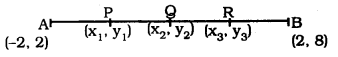Solution:
Let A (-2, 2) = (x1, y1).
B (2, 8) = (x4, y4).
P, Q, R points divide equally the side AB.
Here, we have AP = PQ = QR = RB.
∴ AP : PB = 1 : 3
AQ : QB = 2 : 2 = 1 : 1
∴ AR : RB = 3 : 1.
As per Section formula
i) P divides AB in the ratio 1 : 3ii) Q divides AB in the ratio 2 : 2 means it is mid-point of AB.
∴ As per the Mid-point formula,Q(x2, y2) = (0, 5)

iii) R divides AB in the ratio 3 : 1
m1 = 3, m2 = 1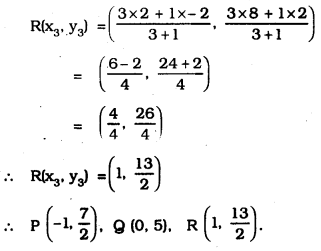Question 10.
Find the area of a rhombus if its vertices are (3, 0), (4, 5), (-1, 4) and (-2, -1) taken in order. [Hint: Area of a rhombus = $$\frac{1}{2}$$ (product of its diagonals]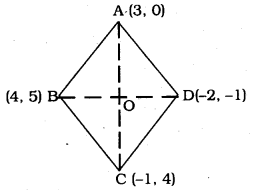Solution:
ABCD is a rhombus.
AC and BD are the diagonals and intersects at ‘O’ and bisects perpendicularly.
∴ Let Diagonal BD = d1 Diagonal AC = d2,We hope the given KSEEB SSLC Class 10 Maths Solutions Chapter 7 Coordinate Geometry Ex 7.2 will help you. If you have any query regarding Karnataka SSLC Class 10 Maths Solutions Chapter 7 Coordinate Geometry Exercise 7.2, drop a comment below and we will get back to you at the earliest

error: Content is protected !!